## 圣诞节购车指南，长安逸动对比日产轩逸

2018年12月25日 14:56 来源：车行天下 超过：14270次关注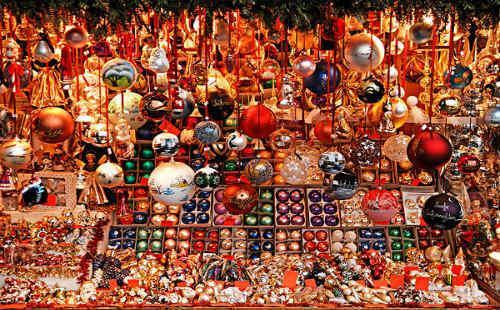其实，类似的事情同样发生在汽车圈。众所周知，由于早年我国本土汽车行业生产力较低，技术发展还处于初级阶段。自主品牌车型往往在很多方面都落后于合资品牌车型。这也导致在很长一段时间以内，合资乃至进口车型在国内汽车市场称王称霸。甚至到了今天仍然有很多人只认可合资车型，对自主品牌车型不屑一顾。实际上，近年来随着我国科学技术的不断创新与进步，自主品牌已然突飞猛进，甚至在很多方面完成了对合资车型的弯道超车，拥有了与其分庭抗礼的能力。接下来，小编就用长安逸动同日产轩逸进行对比，看看如今的自主品牌究竟有哪些可取之处。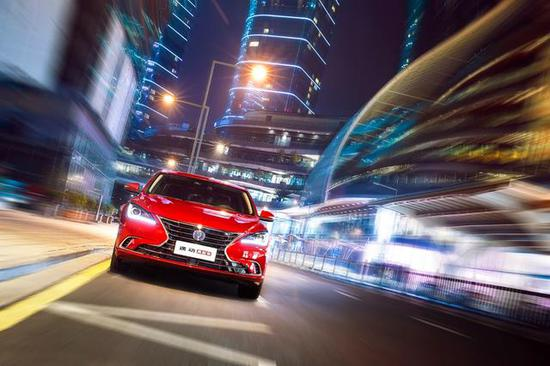（长安逸动）（日产轩逸）

造型时尚 化身潮流风向标

都说眼睛是心灵的窗户，而我们给车型判断第一印象的标准，就是从眼睛得来的，对于当下年轻人来说，很多时候购车真的没有考虑这么多，只要合“眼缘”这项标准达到了，就能让他们下定决心购车。长安逸动作为一款自主品牌自主设计的车型，它的外观，得到了诸多消费者的一致好评。前脸的蝶翼式进气格栅，端庄大气，占据了前脸的很大一部分，同其搭载的LED前大灯相辅相成，看起来锐气四射、动感十足，值得一提的是，长安汽车搭载的这款LED前大灯，内嵌23颗LED芯片并采用厚壁光导技术，明亮却不晃眼，真真是居家旅行必备的实用良品。目光后移，长安逸动还有刀锋式轮毂惹人注目，根根轮辐如刀般出窍，说实话，小编我对这个轮毂爱不释手。长安逸动侧身双腰线也是一个精妙且极富深意的设计，不仅将车身前后两部分完美衔接浑然一体，更是将车身勾勒出一种更加低趴的视觉效果，感觉时刻蓄势待发。在我看来，自主品牌可以做出这样优秀的车型设计，还是非常值得称道的。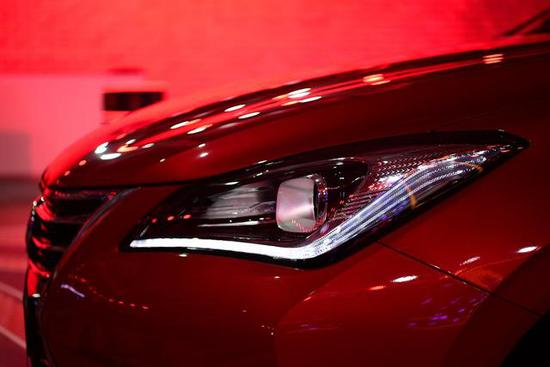（长安逸动LED前大灯）

我们再回过头来再看看日产轩逸这款驰名已久的合资车型。不得不说，这款车的造型设计真的是中规中矩，对比长安逸动前脸的蝶翼式进气格栅，更像是将车灯、进气格栅等元素随意杂糅起来的紧凑面团，看起来虽然不至于被人讨厌，但也确实喜欢不起来。总之在我心里长安逸动在自主设计的造型方面，真的是无懈可击。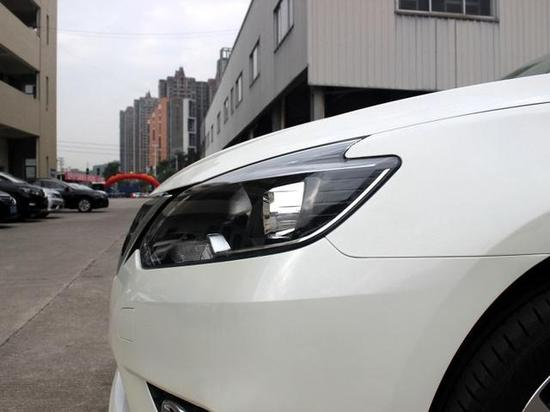（日常轩逸前大灯）

领跑动力 先行一步

长安逸动看起来是一款紧凑型的家用轿车，但实际上在同等级车型中，更像是一款深藏不漏的运动员。长安逸动搭载Blue Core 1.6L GDI缸内直喷全铝合金发动机，功率峰值可达94kW，峰值扭矩可达168N·m。而日产轩逸的发动机功率峰值93 kW，峰值扭矩刚达到154N·m。只要对汽车有一定了解的朋友都应该知道，汽车的功率越大，意味着这辆车可以跑得更快，而扭矩越大，则代表着这辆车的提速越快。日产轩逸作为一款老牌合资车型，却被长安逸动进行了双数据碾压，实在是不得不感叹自主品牌进步真是神速。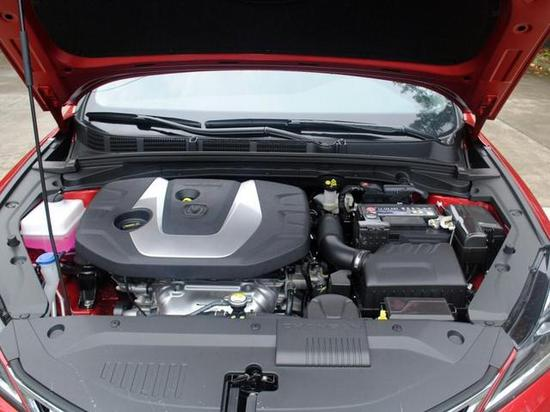（长安逸动发动机）

其实长安逸动还不仅如此，长安逸动在低转速时，能明显感觉到其跃跃欲出的动力，轻点油门就能窜出去的感觉。据称，长安逸动的动力总成根据中国用户使用习惯调校，这款发动机1000转/分时扭矩输出即可达130N•m。可以帮助驾驶者在行驶之初先一步提速，运作更加干脆利落。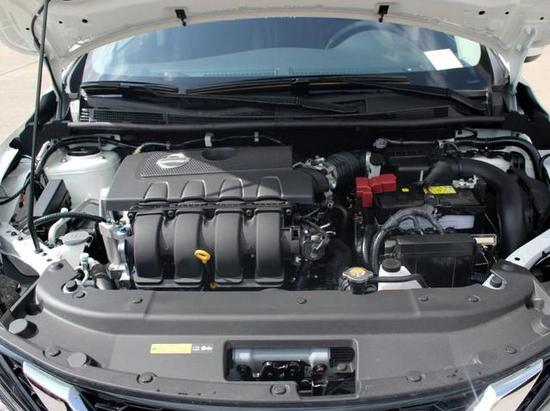（日产轩逸发动机）

价格亲民 12月限时GO

最后我们再将目光放到消费者最关心的价格方面。长安逸动的售价为7.49万—10.69万。对于刚刚开始打拼的年轻人来说，这个价位大部分人是可以承受起的。而同属于紧凑车型的合资车日产轩逸则不这么平易近人，9.98-16.15万元的售价着实让人望而却步。在长安逸动对其进行了颜值、动力、价格三方碾压的情况下，如果仍然无法将你打动，那小编我也只好搬出这个消息：据了解，为祝贺长安汽车用户突破1700万，在这个12月份长安逸动高能来袭，推出“限时GO”促销活动，买车现金直降一万元，还有金融贴息至高4000元、置换补贴至高7000元，这样算下来综合钜惠高达21000元！详情点击：，相信各位可以选择到正确的圣诞礼物~

#### 相关文章

0-500 字已有评论 0条 查看评论>>

### 热门标签

﻿
• 快速找车
• 选择品牌
• 选择品牌
• A  奥迪
• A  阿斯顿·马丁
• A  阿尔法·罗密欧
• B  宝沃
• B  布加迪
• B  巴博斯
• B  保时捷
• B  宾利
• B  奔驰
• B  宝马
• B  本田
• B  别克
• B  标致
• B  比亚迪
• B  宝骏
• B  北汽制造
• B  北汽新能源
• B  北汽幻速
• B  北汽威旺
• B  北京汽车
• B  奔腾
• B  北汽绅宝
• C  长安
• C  长安商用
• C  长城
• C  昌河
• D  大众
• D  道奇
• D  DS
• D  东南
• D  东风风神
• D  东风风行
• D  东风小康
• D  东风风度
• D  东风
• F  福特
• F  丰田
• F  菲亚特
• F  法拉利
• F  福田
• F  福迪
• F  福汽启腾
• G  观致
• G  广汽传祺
• G  广汽吉奥
• G  GMC
• H  红旗
• H  汉腾汽车
• H  哈弗
• H  哈飞
• H  海格
• H  海马
• H  华颂
• H  黄海
• H  华泰
• H  恒天
• J  吉利汽车
• J  捷豹
• J  Jeep
• J  江淮
• J  江铃
• J  金杯
• J  九龙
• J  金旅
• K  凯翼
• K  凯迪拉克
• K  克莱斯勒
• K  科尼塞克
• K  卡威
• K  开瑞
• L  路虎
• L  林肯
• L  劳斯莱斯
• L  兰博基尼
• L  雷克萨斯
• L  铃木
• L  雷诺
• L  理念
• L  力帆
• L  莲花汽车
• L  猎豹
• L  路特斯
• L  陆风
• M  马自达
• M  MG
• M  MINI
• M  玛莎拉蒂
• M  摩根
• M  迈凯轮
• N  纳智捷
• O  欧宝
• O  讴歌
• O  欧朗
• Q  奇瑞
• Q  起亚
• Q  启辰
• R  日产
• R  荣威
• R  瑞麒
• S  三菱
• S  斯威汽车
• S  萨博
• S  smart
• S  斯柯达
• S  斯巴鲁
• S  思铭
• S  双龙
• S  上汽大通
• S  双环
• T  特斯拉
• T  腾势
• W  沃尔沃
• W  五菱汽车
• W  五十铃
• W  威兹曼
• W  威麟
• X  现代
• X  雪佛兰
• X  雪铁龙
• X  西雅特
• Y  一汽
• Y  英菲尼迪
• Y  英致
• Y  依维柯
• Y  野马汽车
• Y  永源
• Z  众泰
• Z  中华
• Z  中兴
• Z  知豆
• 选择车系
• 选择车系
• 车型对比
• 选择品牌
• 选择品牌
• A  奥迪
• A  阿斯顿·马丁
• A  阿尔法·罗密欧
• B  宝沃
• B  布加迪
• B  巴博斯
• B  保时捷
• B  宾利
• B  奔驰
• B  宝马
• B  本田
• B  别克
• B  标致
• B  比亚迪
• B  宝骏
• B  北汽制造
• B  北汽新能源
• B  北汽幻速
• B  北汽威旺
• B  北京汽车
• B  奔腾
• B  北汽绅宝
• C  长安
• C  长安商用
• C  长城
• C  昌河
• D  大众
• D  道奇
• D  DS
• D  东南
• D  东风风神
• D  东风风行
• D  东风小康
• D  东风风度
• D  东风
• F  福特
• F  丰田
• F  菲亚特
• F  法拉利
• F  福田
• F  福迪
• F  福汽启腾
• G  观致
• G  广汽传祺
• G  广汽吉奥
• G  GMC
• H  红旗
• H  汉腾汽车
• H  哈弗
• H  哈飞
• H  海格
• H  海马
• H  华颂
• H  黄海
• H  华泰
• H  恒天
• J  吉利汽车
• J  捷豹
• J  Jeep
• J  江淮
• J  江铃
• J  金杯
• J  九龙
• J  金旅
• K  凯翼
• K  凯迪拉克
• K  克莱斯勒
• K  科尼塞克
• K  卡威
• K  开瑞
• L  路虎
• L  林肯
• L  劳斯莱斯
• L  兰博基尼
• L  雷克萨斯
• L  铃木
• L  雷诺
• L  理念
• L  力帆
• L  莲花汽车
• L  猎豹
• L  路特斯
• L  陆风
• M  马自达
• M  MG
• M  MINI
• M  玛莎拉蒂
• M  摩根
• M  迈凯轮
• N  纳智捷
• O  欧宝
• O  讴歌
• O  欧朗
• Q  奇瑞
• Q  起亚
• Q  启辰
• R  日产
• R  荣威
• R  瑞麒
• S  三菱
• S  斯威汽车
• S  萨博
• S  smart
• S  斯柯达
• S  斯巴鲁
• S  思铭
• S  双龙
• S  上汽大通
• S  双环
• T  特斯拉
• T  腾势
• W  沃尔沃
• W  五菱汽车
• W  五十铃
• W  威兹曼
• W  威麟
• X  现代
• X  雪佛兰
• X  雪铁龙
• X  西雅特
• Y  一汽
• Y  英菲尼迪
• Y  英致
• Y  依维柯
• Y  野马汽车
• Y  永源
• Z  众泰
• Z  中华
• Z  中兴
• Z  知豆
• 选择车系
• 选择车系
• 选择车型
• 选择车型
• 意见反馈